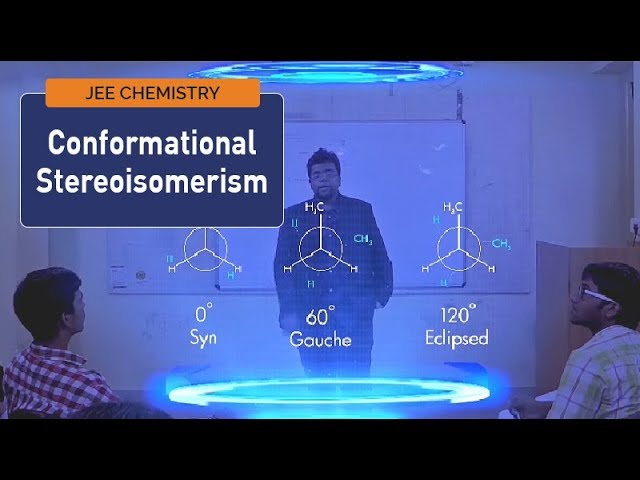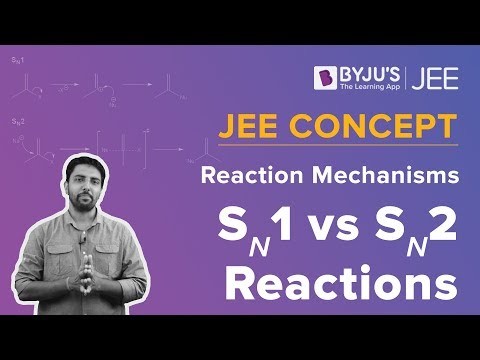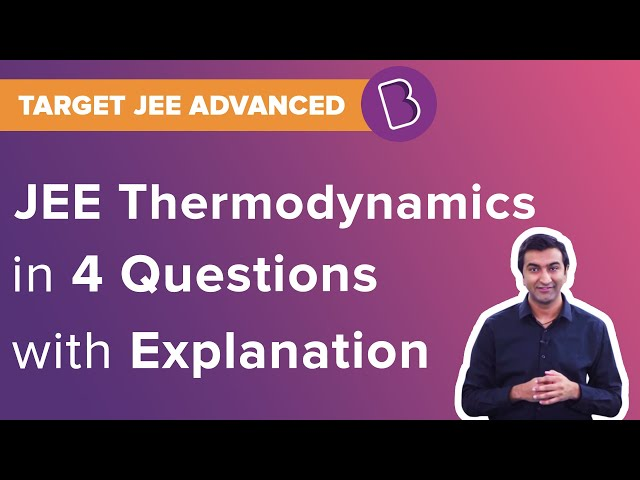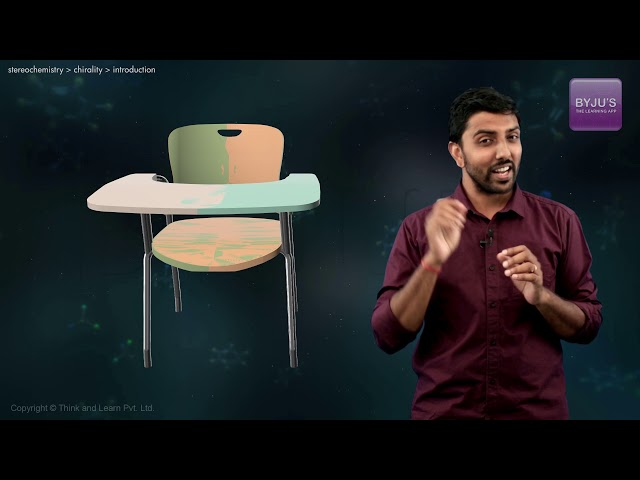Win up to 100% scholarship on Aakash BYJU'S JEE/NEET courses with ABNAT Win up to 100% scholarship on Aakash BYJU'S JEE/NEET courses with ABNAT

# JEE Main 2020 Chemistry Paper With Solutions Jan 9 Shift 2

JEE Main 2020 Jan 9h shift 2 Chemistry paper is provided here and consists of step by step solutions, prepared by our subject specialists. Practising these solutions will help students to improve the speed of solving problems for the JEE Main exam. These solutions are given in a way such that students can easily understand the problems. Students can access the solutions on our web page. It is also available in a PDF format which can be downloaded for free.
January 9 Shift 2 – Chemistry
Question 1. 5 g of Zinc is treated separately with an excess of

I. dilute hydrochloric acid and

II. aqueous sodium hydroxide.

The ratio of the volumes of H2 evolved in these two reactions is:

a) 2:1
b) 1:2
c) 1:1
d) 1:4

Zn + 2NaOH Na2ZnO2 + H2

Zn + 2HCl ZnCl2 + H2

So, the ratio of volume of H2 released in both the cases is 1:1.

Question 2. The solubility product of Cr(OH)3 at 298 K is 6×10-31 . The concentration of hydroxide ions in a saturated solution Cr(OH)3 will be:
a) (18×10-31)1/4
b) (18×10-31)1/2
c) (2.22×10-31)1/4
d) (4.86×10-29)1/4

Cr(OH)3 (s) Cr3+(aq) + 3OH(aq)

1-S S 3S

Ksp = 27S4

6×10-31 = 27S4

S = [(6/27)×10-31]1/4

[OH] = 3S = 3×[(6/27)×10-31]1/4

= (18×10-31)1/4 M

Question 3. Among the statements (a)-(d), the correct ones are:

a) Lithium has the highest hydration enthalpy among the alkali metals.

b) Lithium chloride is insoluble in pyridine.

c) Lithium cannot form ethynide upon its reaction with ethyne.

d) Both lithium and magnesium react slowly with H2O.

a) (a), (b) and (d) only
b) (b) and (c) only
c) (a), (c) and (d) only
d) (a) and (d) only

Only LiCl amongst the first group chlorides dissolve in pyridine because the solvation energy of lithium is higher than the other salts of the same group. Lithium does not react with ethyne to form ethynilide due to its small size and high polarizability. Lithium and Magnesium both have very small sizes and very high ionization potentials so, they react slowly with water. Amongst all the alkali metals, Li has the smallest size hence, the hydration energy for Li is maximum.

Question 4. The first and second ionization enthalpies of a metal are 496 and 4560 kJ mol-1 respectively. How many moles of HCl and H2SO4, respectively, will be needed to react completely with 1 mole of metal hydroxide?
a) 1 and 2
b) 1 and 0.5
c) 1 and 1
d) 2 and 0.5

The given data for ionization energies clearly shows that IE2 ≫ IE1. So, the element belongs to the first group. Therefore, we can say that this element will be monovalent and hence forms a monoacidic base of the type MOH.

MOH + HCl MCl + H2O

2MOH + H2SO4 M2SO4 + 2H2O

So, from the above equation we can say that, 1 mole of metal hydroxide requires 1 mole of HCl and 0.5 mole of H2SO4.

Question 5. In the figure shown below reactant A (represented by the square) is in equilibrium with product B (represented by circle). The equilibrium constant is: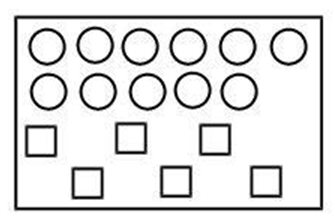a) 1
b) 2
c) 8
d) 4

Let us assume the equation to be A ⇌ B,

Number of particles of A = 6

Number of particles of B = 11

K= 11/6 ≈ 2

Question 6. The correct order spin-only magnetic moments of the following complexes is:

I. [Cr(H2O)6]Br2

II. Na4[FeCN6]

III. Na3[Fe(C2O4)3] (0 > P)

IV. (Et4N)2[CoCl4]

a) (III)>(I)>(II)>(IV)
b) (III)>(I)>(IV)>(II)
c) (I)>(IV)>(III)>(II)
d) (II)≈(I)>(IV)>(III)

Complex (I) has the central metal ion as Fe2+ with strong field ligands.

Configuration of Fe2+ = [Ar] 3d6

Strong field ligands will pair up all the electrons and hence the magnetic moment will be zero.

Complex (II) has the central metal ion as Cr2+ with weak field ligands.

Configuration of Cr2+ = [Ar] 3d4

As weak field ligands are present, pairing does not take place. There will be 4 unpaired electrons and hence the magnetic moment = √24 B.M.

Complex (III) has the central metal ion as Co2+ with weak field ligands.

Configuration of Co2+ = [Ar] 3d7

As weak field ligands are present no pairing can occur. There will be 3 unpaired electrons and hence the magnetic moment = √15 B.M.

Complex (IV) has the central metal ion as Fe3+ with strong field ligands.

Configuration of Fe3+ =[Ar] 3d5

Strong field ligands will pair up the electrons but as we have a [Ar] 3d5 configuration, one electron will remain unpaired and hence the magnetic moment will be √3 B.M.

Question 7. The true statement amongst the following.
a) S is a function of temperature but S is not a function of temperature.
b) Both S and S are functions of temperature.
c) Both S and S are not functions of temperature.
d) S is not a function of temperature but S is a function of temperature.

Entropy is a function of temperature, at any temperature, the entropy can be given as:

$$\begin{array}{l}S_{T} = \int_{0}^{T}\frac{nCdT}{T}\end{array}$$

Change in entropy is also a function of temperature, at any temperature, the entropy change can be given as:

$$\begin{array}{l}\Delta S = \int \frac{dq}{T}\end{array}$$

Question 8. The reaction of H3N3B3Cl3 (A) with LiBH4 in tetrahydrofuran gives inorganic benzene (B). Furthur, the reaction of (A) with (C) leads to H3N3B3(Me)3. Compounds (B) and (C) respectively, are:
a) Boron nitride, MeBr
b) Diborane, MeMgBr
c) Borazine, MeBr
d) Borazine, MeMgBr

H3N3B3Cl3 + LiBH4 B3N3H6 + LiCl + BCl3

B3N3H3Cl3 + 3CH3MgBr B3N3H3(CH3)3 + 3MgBrCl

So, we can say that,

B is B3N3H6 and C is CH3MgBr

Question 9. A mixture of gases O2, H2 and CO are taken in a closed vessel containing charcoal. The graph that represents the correct behaviour of pressure with time is: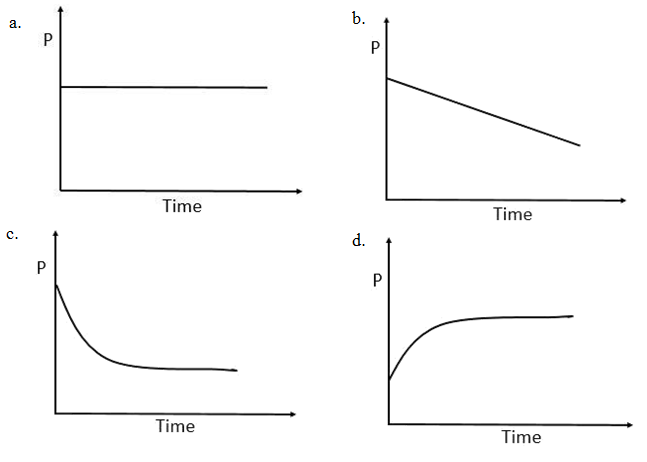As H2,O2 and CO gets adsorbed on the surface of charcoal, the pressure decreases. So, option (a) and (d) can be eliminated. After some time, as almost all the surface sites are occupied, the pressure becomes constant.

Question 10. The isomer(s) of [Co(NH3)4Cl2] that has/have a Cl-Co-Cl angle of 900, is/are:
a) cis only
b) trans only
c) meridional and trans
d) cis and trans

In cis-isomer, similar ligands are at an angle of 900.

Question 11.Amongst the following, the form of water with lowest ionic conductance at 298 K is:
a) distilled water
b) sea water
c) saline water used for intra venous injection
d) water from a well

In distilled water there are no ions present except H+ and OH ions, both of which are immensely minute in concentration, that renders their collective conductivity negligible.

Question 12. The number of sp2 hybrid orbitals in molecule of benzene is:
a) 18
b) 24
c) 6
d) 12

Benzene (C6H6) has 6 sp2 hybridized carbons. Each carbon has 3 -bonds and 1 -bond. 3 -bonds means that there are 3 sp2 hybrid orbitals for each carbon. Hence, the total number of sp2 hybrid orbitals is 18.

Question 13. Which of the following reactions will not produce a racemic product?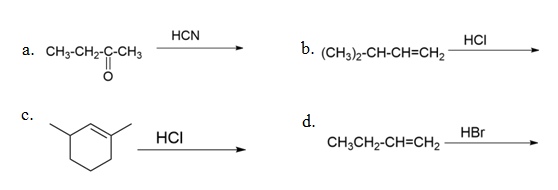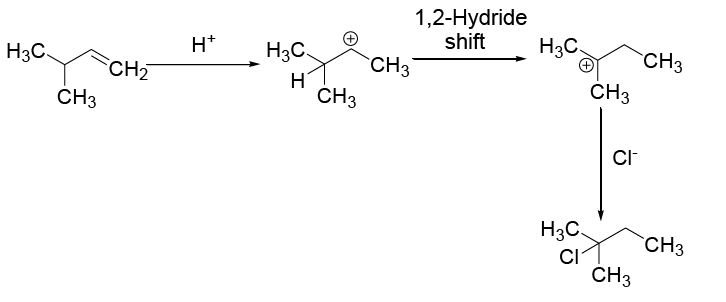Question 14. Which of the following has the shortest C-Cl bond?
a) Cl- CH=CH2
b) Cl-CH=CH-CH3
c) Cl-CH=CH-OCH3
d) Cl-CH=CH-NO2

There is extended conjugation present in option (d), which will reduce the length of C-Cl bond to the greatest extent which can be represented as follows: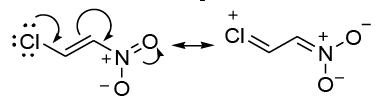Question 15.Biochemical oxygen demand (BOD) is the amount of oxygen required (in ppm):

a) for the photochemical breakdown of waste present in 1m3 volume of a water body.
b) by anaerobic bacteria to break-down inorganic waste present in a water body.
c) by bacteria to break-down organic waste in a certain volume of water sample.
d) for sustaining life in a water body.

Biochemical oxygen demand (BOD) is the amount of dissolved oxygen used by microorganisms in the biological process of metabolizing organic matter in water.

Question 16. Which polymer has chiral, monomer(s)?
a) Buna-N
b) Neoprene
c) Nylon 6,6
d) PHBV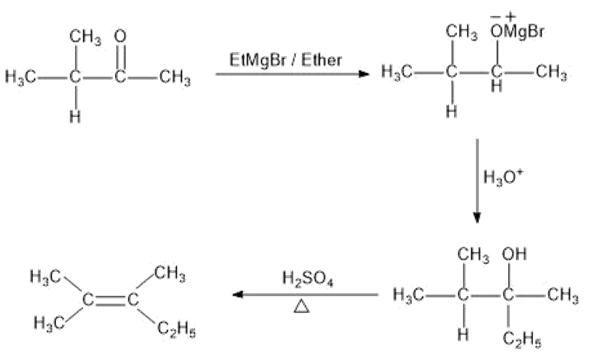Question 17. A, B and C are three biomolecules. The results of the tests performed on them are given below:

 Molisch’s Test Barfoed Test Biuret Test A Positive Negative Negative B Positive Positive Negative C Negative Negative Positive

A, B and C are respectively

a) A = Lactose B = Glucose C = Albumin
b) A = Lactose B = Glucose C = Alanine
c) A = Lactose B = Fructose C = Alanine
d) A = Glucose B = Sucrose C = Albumin

Lactose, glucose and fructose gives positive Molisch’s test.

Glucose gives positive Barfoed’s test whereas sucrose gives a negative for Barfoed’s test.

Albumin gives positive for Biuret test whereas alanine gives a negative Biuret test.

Question 18. The decreasing order of basicity of the following amines is: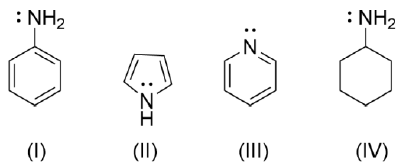a) I > II > III > IV
b) IV > III > I > II
c) II > I > III > IV
d) IV > I > II > III

The basicity of the compound depends on the availability of the lone pairs.

In compound IV, Nitrogen is sp3 hybridized.

In compound III, Nitrogen is sp2 hybridized and the lone pairs are not involved in resonance.

In compound I, Nitrogen is sp2 hybridized and the lone pairs are involved in resonance.

In compound II, Nitrogen is sp2 hybridized and the lone pairs are involved in resonance such that, they are contributing to the aromaticity of the ring. From the above points we can conclude that the basicity order should be IV > III > I > II.

Question 19. The compound [P] is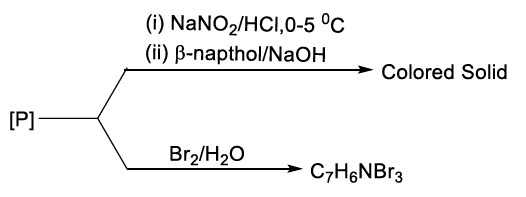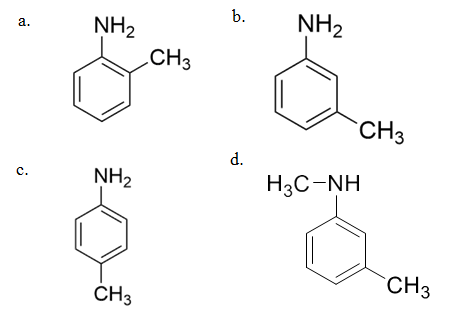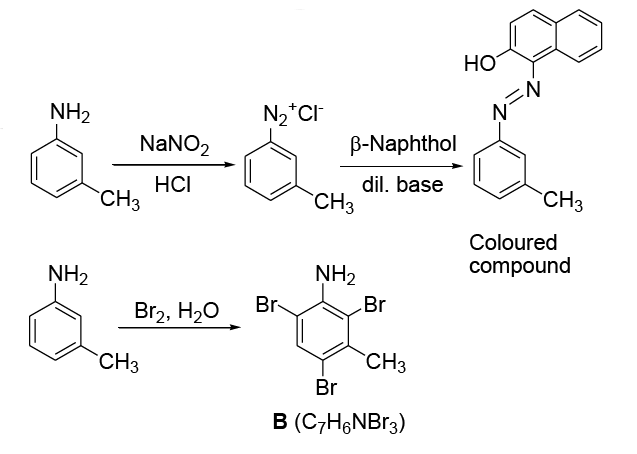Question 20. In the following reaction A is :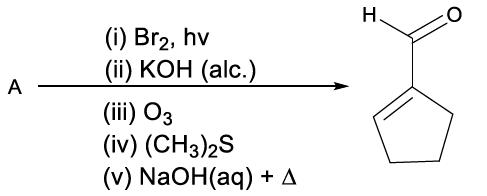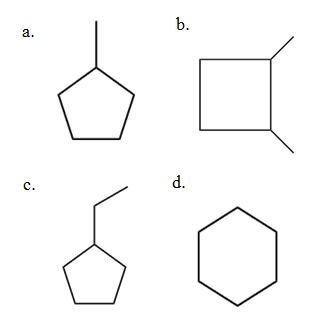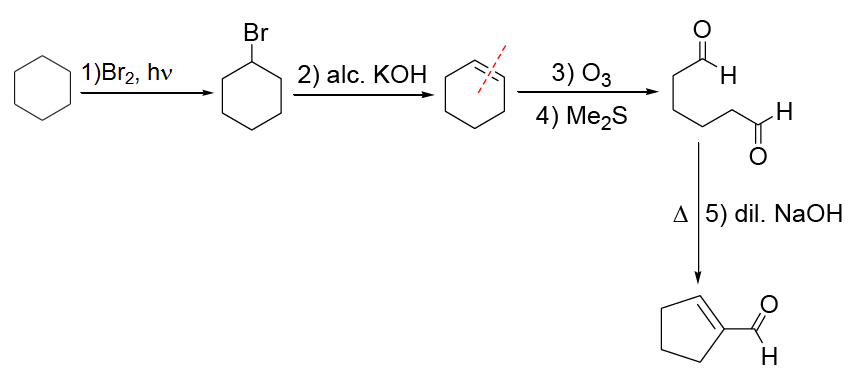Question 21. The sum of total number of bonds between chromium and oxygen atoms in chromate and dichromate ions is: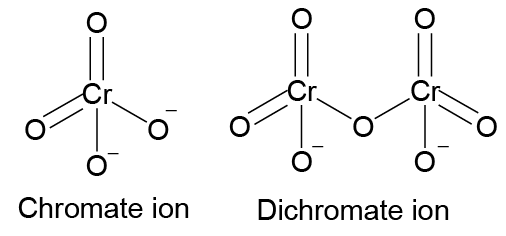Question 22.A sample of milk splits after 60 min. at 300K and after 40 min at 400K when the population of lactobacillus acidophilus in it doubles . The activation energy (in kJ/mol) for this process is closest to : (Given, R = 8.3 J mol-1K-1), ln(2/3) = 0.4, e-3 = 4.0)

The generation time can be utilized to get an indication of the rate ratio. Let the amount generated be (x).

Rate = Amount generated/Time taken

Rate300 K = (x)/60

Rate400 K =(x)/40

Rate 300K/Rate 400K = 40/60

For the same concentration (which is applicable here), the rate ratio can also be equal to the ratio of rate constants.

$$\begin{array}{l}ln\left [ \frac{K_{at \: 400K}}{K_{at \: 300K}} \right ] = \frac{E_{a}}{R}\left [ \frac{1}{T_{1}}-\frac{1}{T_{2}} \right ]\end{array}$$
$$\begin{array}{l}ln\frac{60}{40} = \frac{E_{a}}{8.3}\left [ \frac{1}{300}-\frac{1}{400} \right ]\end{array}$$

Ea = 0.4 × 8.3 × 1200 = 3984 J/mol = 3.98 kJ/mol

Question 23. One litre of sea water (d =1.03g/cm3) contains 10.3 mg of O2 gas. Determine the concentration of O2 in ppm:

Ppm = (WSolute/W Solution)×100

Using the density of the solution and its volume (1L = 1000 mL = 1000 cm3), the weight of the solution can be calculated.

Wsolution = 1.03 × 1000 = 1030 g

Thus, ppm = ((10.3 x 10 -3g)/1030 g) × 100

Question 24. A cylinder containing an ideal gas (0.1 mol of 1.0 dm3 ) is in thermal equilibrium with a large volume of 0.5 molal aqueous solution of ethylene glycol at it freezing point. If the stoppers S1 and S2 (as shown in the figure) suddenly withdrawn, the volume of the gas in liters after equilibrium is achieved will be: (Given, Kf (water) = 2.0 K kg mol-1 ,R = 0.08 dm3 atm K-1 mol-1)

Kf = 2

Molality, ‘m’ = 0.5

Tf = Kf × m

= (0.5 × 2) = 1

So, the initial temperature now becomes 272 K. Further using the given value of moles and initial volume of the gas and the calculated initial temperature value, we can find out the initial pressure of the ideal gas contained inside the piston.

Pgas = nRT/V1

= (0.1)(0.08)(272) = 2.176 atm

Now, on releasing the piston against an external pressure of 1 atm, the gas will expand until the final pressure of the gas, i.e. P2 becomes equal to 1 atm. During this expansion, since no reaction is happening and the temperature of the gas is not changing as well, the boyle’s law relation can be applied.

P1V1 = P2V2

2.176 × 1 = 1 × V2

V2 = 2.716

Question 25. Consider the following reactions;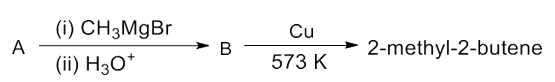The mass percentage of carbon in A is: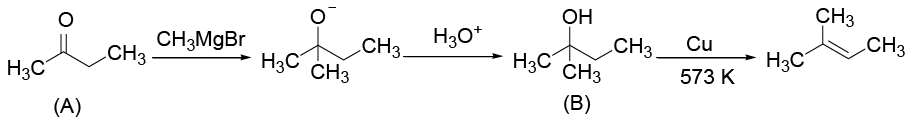Compound A is CH3(CO)CH2CH3 (C4H8O)

The percentage of carbon in compound A by weight is WCarbon/WCompound = 12×4/72 = 66.67